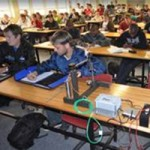FEA Assignment

For this assignment, you need to complete the form (link on image) below which requires you to do the following:

1. Interpret the test setup and volunteer measurements and report the values you assumed to be correct.
2. Interpret the test results and provide the requested values.
3. Complete a hand calc using the measurements and assuming a clamped cantilevered beam with a point load near the tip and provide the required results.
4. Represent the structure (as tested and calculated by hand) using FEA 1D beam elements and provide the required results.
5. Repeat the above using FEA 2D shell elements.
6. Repeat the above using FEA 3D Tetrahedral elements
7. Repeat the above using FEA 3D hexahedral elements.

Click the image below to access the assignment form where you need to submit all the required results:Resources

The links below show the steps needed in the various software packages to build the FEA models required to complete the assignment.